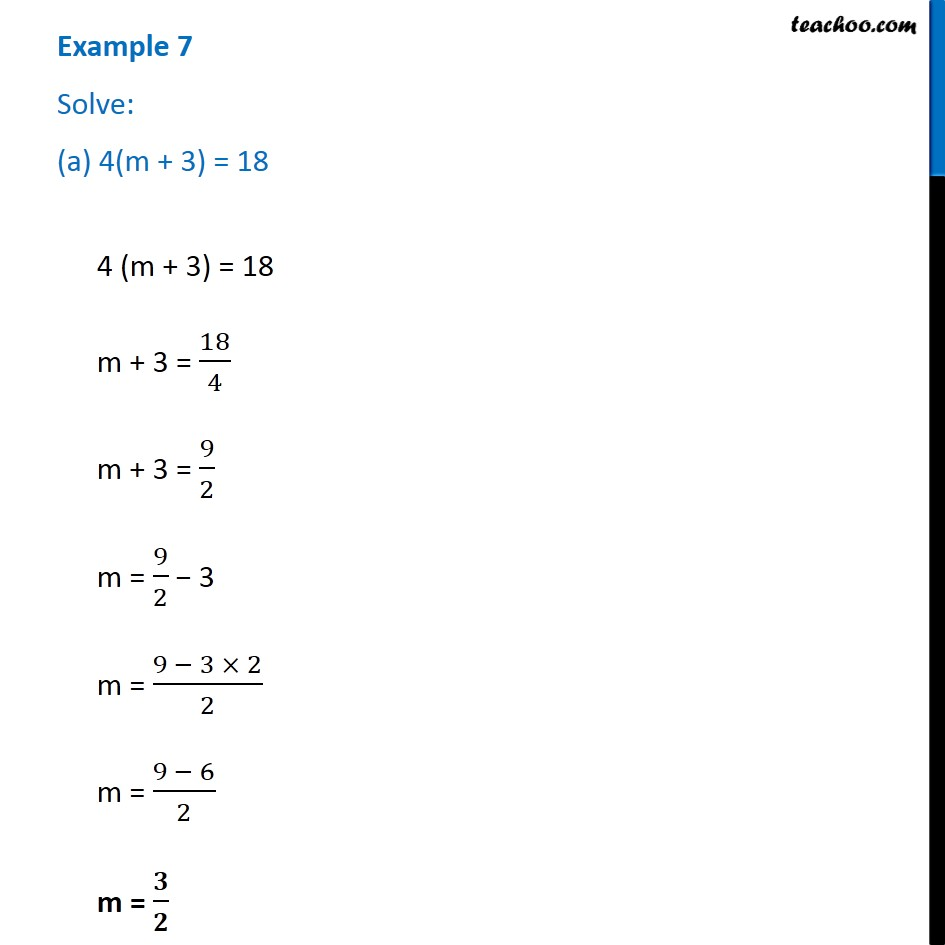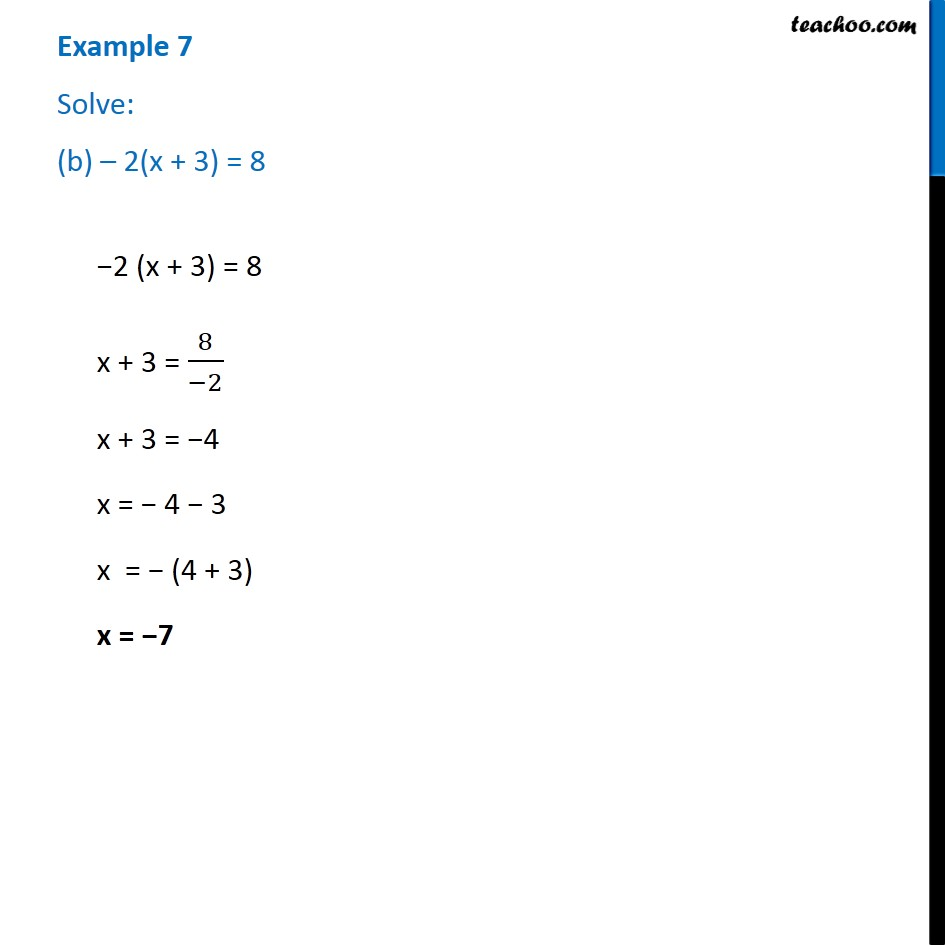1. Chapter 4 Class 7 Simple Equations
2. Serial order wise
3. Examples

Transcript

Example 7 Solve: (a) 4(m + 3) = 184 (m + 3) = 18 m + 3 = 18/4 m + 3 = 9/2 m = 9/2 − 3 m = (9 − 3 × 2)/2 m = (9 − 6)/2 m = 𝟑/𝟐 Example 7 Solve: (b) – 2(x + 3) = 8−2 (x + 3) = 8 x + 3 = 8/(−2) x + 3 = −4 x = − 4 − 3 x = − (4 + 3) x = −7

Examples